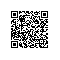# 《数值分析（原书第2版）》—— 0.5　微积分回顾

### 0.5　微积分回顾

+f(k)(x0)k!(x-x0)k+f(k+1)(c)(k+1)!(x-x0)k+1　　结果的多项式部分，x-x0构成的最高k阶项，被称为以x0为中心的函数f的k阶泰勒多项式.最后一项称为泰勒余项.当泰勒余项足够小时，泰勒定理给出了一般的平滑函数的多项式近似.这对于计算机求解问题十分方便，如前所述，多项式的求值计算非常高效.　　例0.9　对于中心在x0=0的20
～
21f（x）=sinx找出一个4阶泰勒多项式P4(x).计算使用P4(x)估计sinx时的最大可能的误差，其中x≤0.0001.

1.使用中值定理证明存在0(a) f(x)=x3-4x+1(b) f(x)=5cosπx-4(c) f(x)=8x4-8x2+1
2.在区间［0，1］上找到函数f（x）满足均值定理的数字c.
(a) f(x)=ex(b) f(x)=x2(c) f(x)=1/(x+1)
3.在区间［0，1］上找到对于函数f（x）、g（x）满足积分均值定理的数字c.
(a) f(x)=x,g(x)=x(b) f(x)=x2,g(x)=x(c) f(x)=x,g(x)=ex
4.为以下函数找到x=0时的二阶泰勒多项式:
(a) f(x)=ex2(b) f(x)=cos5x(c) f(x)=1/(x+1)
5.为以下函数找到x=0时的5阶泰勒多项式:
(a) f(x)=ex2(b) f(x)=cos2x(c) f(x)=ln(1+x)(d) f(x)=sin2x
6.（a） 在点x=1找到函数f(x)=x-2的4阶泰勒多项式.
（b） 利用（a）的结果近似f（0.9）和f（1.1）.
（c） 使用泰勒余项确定泰勒多项式的误差公式.给出（b）部分两个近似的误差上界.（b）部分中的哪一个近似更加接近正确的值？
（d） 使用计算器比较每个问题中实际误差和（c）部分中自己计算的误差上界.
7.对于函数f（x）=lnx完成习题6的（a）~（d）.
8.（a） 找出中心在x=0的函数f（x）=cosx的5阶泰勒多项式.
（b） 找到在区间［-π/4，π/4］上使用P（x）近似函数f（x）=cosx的误差上界.
9.当x足够小时，对于1+x的一个常见近似是1+12x.使用函数f(x)=1+x的1阶泰勒多项式的余项确定形如1+x=1+12x±E的公式.计算在近似1.02时对应的E.使用计算器比较实际的误差和估计的误差界E.22